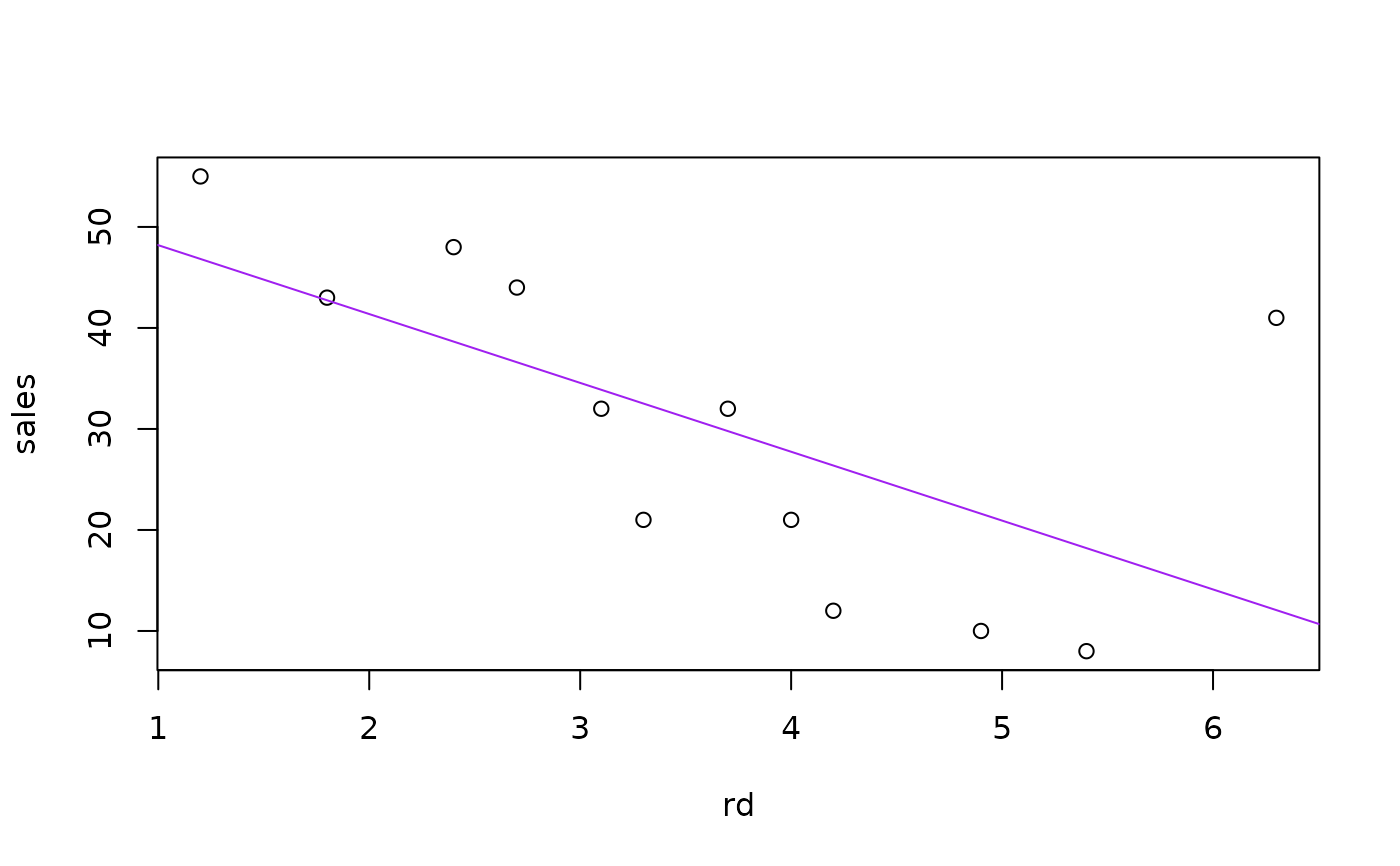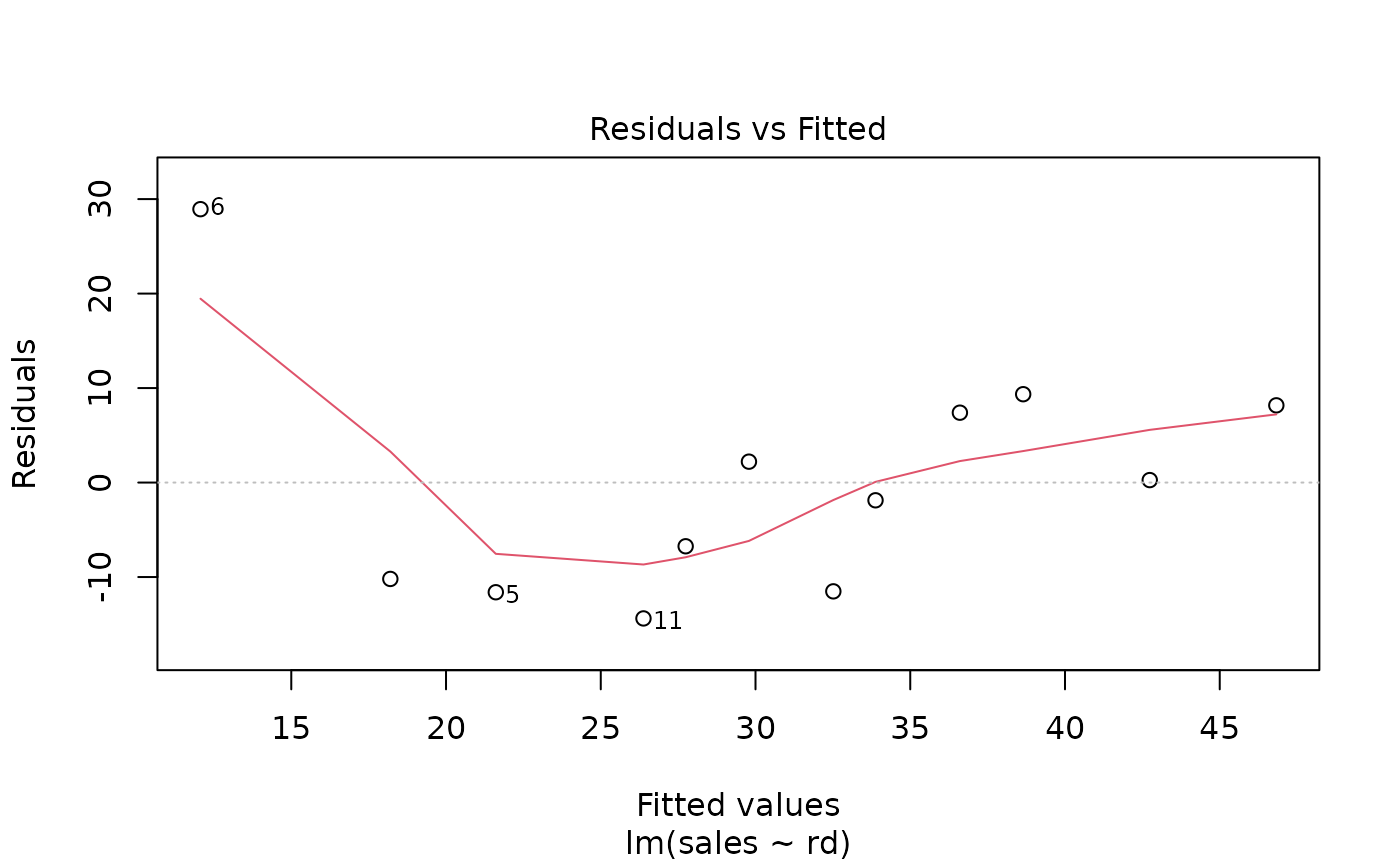Data for Exercise 9.36 and Example 9.8

Randd

## Format

A data frame/tibble with 12 observations on two variables

rd

research and development expenditures (in million dollars)

sales

sales (in million dollars)

## Examples


plot(sales ~ rd, data = Randd)
model <- lm(sales ~ rd, data = Randd)
abline(model, col = "purple")summary(model)
#>
#> Call:
#> lm(formula = sales ~ rd, data = Randd)
#>
#> Residuals:
#>      Min       1Q   Median       3Q      Max
#> -14.3800 -10.5291  -0.8083   7.5896  28.9340
#>
#> Coefficients:
#>             Estimate Std. Error t value Pr(>|t|)
#> (Intercept)   55.008     10.126   5.433 0.000288 ***
#> rd            -6.816      2.626  -2.596 0.026690 *
#> ---
#> Signif. codes:  0 ‘***’ 0.001 ‘**’ 0.01 ‘*’ 0.05 ‘.’ 0.1 ‘ ’ 1
#>
#> Residual standard error: 12.95 on 10 degrees of freedom
#> Multiple R-squared:  0.4025,	Adjusted R-squared:  0.3428
#> F-statistic: 6.737 on 1 and 10 DF,  p-value: 0.02669
#>
plot(model, which = 1)rm(model)